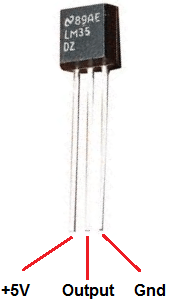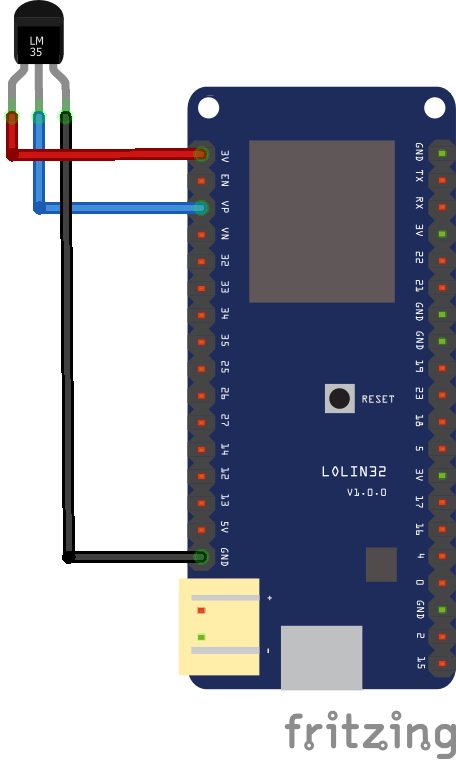Home » ESP32 and LM35 temperature sensor

# ESP32 and LM35 temperature sensor

In this example we will connect an LM35 temperature sensor to our ESP32 module

The LM35 series are precision integrated-circuit temperature sensors, whose output voltage is linearly proportional to the Celsius (Centigrade) temperature. The LM35 thus has an advantage over linear temperature sensors calibrated in Kelvin, as the user is not required to subtract a large constant voltage from its output to obtain convenient Centigrade scaling. The LM35 does not require any external calibration or trimming to provide typical accuracies of ±1/4°C at room temperature and ±3/4°C over a full -55 to +150°C temperature range

Here is a picture of the pins, its important to get these correct or you can damage the sensor, although its stated 5v in the image below we never had any issue connecting this to 3v3Schematics

Very simple to connect Vcc is 3v3, Gnd is any Gnd and out goes to ESp32 LOLIN 32 A0, you can see this belowCode

[cpp]

int RawValue= 0;
double Voltage = 0;
double tempC = 0;
double tempF = 0;

void setup(){
Serial.begin(9600);
}

void loop(){

Voltage = (RawValue / 2048.0) * 3300; // 5000 to get millivots.
tempC = Voltage * 0.1;
tempF = (tempC * 1.8) + 32; // conver to F
Serial.print(“Raw Value = ” ); // shows pre-scaled value
Serial.print(RawValue);
Serial.print(“\t milli volts = “); // shows the voltage measured
Serial.print(Voltage,0); //
Serial.print(“\t Temperature in C = “);
Serial.print(tempC,1);
Serial.print(“\t Temperature in F = “);
Serial.println(tempF,1);
delay(500);
}

[/cpp]

Results

Here are the results via the serial monitor

Raw Value = 173 milli volts = 279 Temperature in C = 27.9 Temperature in F = 82.2
Raw Value = 173 milli volts = 279 Temperature in C = 27.9 Temperature in F = 82.2
Raw Value = 170 milli volts = 274 Temperature in C = 27.4 Temperature in F = 81.3
Raw Value = 173 milli volts = 279 Temperature in C = 27.9 Temperature in F = 82.2
Raw Value = 169 milli volts = 272 Temperature in C = 27.2 Temperature in F = 81.0
Raw Value = 168 milli volts = 271 Temperature in C = 27.1 Temperature in F = 80.7
Raw Value = 172 milli volts = 277 Temperature in C = 27.7 Temperature in F = 81.9
Raw Value = 169 milli volts = 272 Temperature in C = 27.2 Temperature in F = 81.0